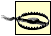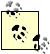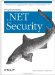# 14.4 Extending the .NET Framework

In this section, we extend the .NET Framework by implementing the Extended Tiny Encryption Algorithm (XTEA). We have selected XTEA because it is simple to express, allowing us to concentrate on the details of adding a new algorithm rather than the specifics of the algorithm itself. Most symmetric encryption algorithms rely on large lookup tables that speed up encryption, but these are not suitable for listing in this book.

We have provided only a C# implementation of the XTEA algorithm. Like almost all encryption algorithms, XTEA relies on using bitwise shift operations on unsigned data types; this is not possible in Visual Basic .NET without creating additional support functions to compensate for the limited numeric support Visual Basic .NET provides.

#### 14.4.1 The Extended Tiny Encryption Algorithm Explained

The Tiny Encryption Algorithm (TEA) was developed by David Wheeler and Roger Needham of Cambridge University, England. They designed TEA to be simple, quick, and easily expressed in a range of programming languages. Wheeler and Needham released the XTEA in response to a weakness discovered in the original algorithm. Since that time, further weaknesses have emerged with TEA, leading the designers to make more modifications (often referred to as XTEA).

To complicate matters, poor documentation has made differing implementations incompatible. This confusion has restricted the adoption of XTEA, and it is not frequently used. There has been little analysis of the XTEA algorithm, and the security of the design is unknown.We have selected the XTEA algorithm for clarity, not security. We recommend that you do not use this implementation to protect confidential data.

#### 14.4.2 Defining the Abstract Class

First, create an abstract class representing the XTEA algorithm. The abstract class extends the System.Security.Cryptography.SymmetricAlgorithm class and is the foundation for our managed code implementation:

`using System.Security.Cryptography; public abstract class XTEA : SymmetricAlgorithm { }`

You have created the abstract class to conform to the .NET class model, allowing you to add other XTEA implementations in the future. You can omit the abstract class, but we feel that the extra flexibility of this model is well worth the effort.

#### 14.4.3 Defining the Implementation Class

The SymmetricAlgorithm class has two roles: the first is to describe an algorithm and the second is to provide a mechanism for creating instances of ICryptoTransform to encrypt or decrypt data. Table 14-8 lists the SymmetricAlgorithm-protected fields, each of which, with the exception of LegalKeySizesValue and LegalBlockSizesValue, can also be get and set using a corresponding property; each property holds data that describes an aspect of the encryption algorithm configuration.

##### Table 14-8. The protected fields of the SymmetricAlgorithm class

Name

Data type

Description

`BlockSizeValue`
`int`

The cipher function block size in bits

`FeedbackSizeValue`
`int`

The number of data bits fed back into the cipher function in chained cipher modes

`IVValue`
`byte[]`

The value of the initialization vector

`KeySizeValue`
`int`

The size of the secret key in bits

`KeyValue`
`byte[]`

The value of the secret key

`LegalBlockSizeValue`
`KeySize`

The range of data block sizes that can be processed by the cipher function

`LegalKeySizeValue`
`KeySize`

The range of key sizes supported by the algorithm

`ModeValue`
`CipherMode`

Represents the cipher mode

`PaddingValue`
`PaddingMode`

Specifies the technique to use in padding incomplete data blocks

We define the XTEAManaged class as our implementation of the XTEA algorithm. The XTEA cipher operates on 64-bit blocks of data and uses a 128-bit secret key. Use the class constructor to set these values, implementing the LegalKeySizeValue and LegalBlockSizeValue fields:

`using System.Security.Cryptography; public class XTEAManaged : XTEA {     public XTEAManaged(  ) {         // define the valid block and key sizes         LegalKeySizesValue   = new KeySizes[] {new KeySizes(128, 128, 0)};         LegalBlockSizesValue = new KeySizes[] {new KeySizes(64, 64, 0)};     }`

The GenerateKey and GenerateIV methods are marked as abstract in the SymmetricAlgorithm class; therefore, they must be included in the implementation class; these methods must create a random secret key or initialization vector that is subject to the design of the algorithm. Create a 128-bit secret key and a 64-bit IV using the random number generator; see Chapter 17 for more information about creating and managing keys.

`    public override void GenerateIV(  ) {         // create the PRNG         RandomNumberGenerator x_random = RandomNumberGenerator.Create(  );         // create the data array that will hold the IV         IVValue = new byte;         x_random.GetBytes(IVValue);     }     public override void GenerateKey(  ) {         // create the PRNG         RandomNumberGenerator x_random = RandomNumberGenerator.Create(  );         // create the data array that will hold the IV         KeyValue = new byte;         x_random.GetBytes(KeyValue);     }     public override ICryptoTransform CreateDecryptor(byte[] p_key, byte[] p_iv) {         return new XTEAManagedDecryptor(p_key);     }     public override ICryptoTransform CreateEncryptor(byte[] p_key, byte[] p_iv) {         return new XTEAManagedEncryptor(p_key);     } }`

The remaining methods create implementations of the ICryptoTransform interface, called XTEAManagedEncryptor and XTEAManagedDecryptor. Notice that you do not pass the p_iv parameter into the constructor for the ICryptoTranform implementations; this is because our XTEA implementation supports only the ECB cipher mode; therefore, you do not need to use an IV as a seed value for cipher function chaining.

#### 14.4.4 Defining an Abstract Transformation

We have defined an abstract class that you used as the basis for the ICryptoTransform implementation classes. The main purpose of this class is to provide a common location for utility methods and ICryptoTransform interface members that are the same in both the encryption and decryption classes:

`using System; using System.Security.Cryptography; public abstract class XTEAManagedAbstractTransform : ICryptoTransform {     protected uint[] o_key;     // the key, expressed as a series of 32bit words     public XTEAManagedAbstractTransform(byte[] p_key) {         // convert the key from an array of bytes into         // and array of unsigned 32-bit words, as required         // by the XTEA specification         o_key = ConvertBytesToUints(p_key, 0, p_key.Length);        }`

The constructor simply converts the secret key from a byte array, as expressed by the .NET Framework, to an array of unsigned 32-bit integers as required by the XTEA algorithm; store the key as an instance array. The XTEA algorithm has been designed with unsigned 32-bit integers in mind, and you can use the ConvertBytesToUints and ConvertUintstoBytes methods in the encryption and decryption transformations to convert to and from the byte representation that the .NET Framework uses:

`    protected uint[] ConvertBytesToUints(byte[] p_data, int p_offset,      int p_count) {         // allocate an array - each uint requires 4 bytes         uint[] x_result = new uint[p_count/4];         // run through the data and create the unsigned ints from         // the array of bytes         for (int i = p_offset, j = 0; i < p_offset + p_count; i+=4, j++) {             x_result[j] = BitConverter.ToUInt32(p_data, i);         }         // return the array of 32-bit unsigned ints         return x_result;     }     protected byte[] ConvertUintstoBytes(uint[] p_data) {         // convert the unit[] into a byte[]         byte[] x_result = new byte[p_data.Length * 4];         // run through the data and create the bytes from         // the array of unsigned ints         for (int i = 0, j = 0; i < p_data.Length; i++, j+=4) {             byte[] x_interim = BitConverter.GetBytes(p_data[i]);             Array.Copy(x_interim, 0, x_result, j, x_interim.Length);         }         // return the array of 8-bit bytes         return x_result;     }`

The ICryptoTransform interface requires the following properties, which describe the algorithm used by the transformation. Some encryption algorithms (for example, Rijndael/AES) support a range of block and key sizes, and it can be useful to examine the settings for a specific transformation, especially if the SymmetricAlgorithm instance used to create it is not available.

The XTEA algorithm accepts and produces 64-bit blocks of data, and so the InputBlockSize and OutputBlockSize properties both return "8", representing the number of bytes note that unlike the SymmetricAlgorithm class, the ICryptoTransform interface expresses blocks in bytes rather than bits.

The CryptoStream class uses the CanTransformMultipleBlocks property when breaking up data for processing. If this property returns true, then the implementation of the TransformBlock method will process data arrays that are multiples of InputBlockSize. The implementations of XTEA processes only 64-bit blocks, and for simplicity, return false from this method, indicating that CryptoStream should only request processing for data in 64-bit blocks and not multiples thereof. Set the CanReuseTransform property always to return false so that our XTEA transformation can be used only to process one set of data to either encrypt a single plaintext or decrypt a single ciphertext:

`    public bool CanReuseTransform {         get {             return false;         }     }     public bool CanTransformMultipleBlocks {         get {             return false;         }     }     public int InputBlockSize {         get {             return 8;         }     }     public int OutputBlockSize {         get {             return 8;         }     }`

The remaining members of this class are included simply to comply with the interface requirements; the Dispose method is useful if your algorithm uses unmanaged code (for accessing specialized acceleration hardware or key readers), but the XTEA implementation is written using only managed code; therefore you have no need to use statements to clean up resources. The definitions for TransformBlock and TransformFinalBlock are abstract, because they represent the point of specialization for the encryption and decryption transformations:

`    public virtual void Dispose(  ) {         // we use no unmanaged resources     }     public abstract int TransformBlock(byte[] p_buffer, int p_offset,          int p_count, byte[] p_output, int p_output_offset);     public abstract byte[] TransformFinalBlock(byte[] p_buffer, int p_offset,          int p_count); }`

#### 14.4.5 Defining the Encryption Transformation

The XTEAManagedEncryptor class is instantiated by the XTEAManaged.CreateEncryptor method (see Section 14.4.2) and is used to transform blocks of plaintext into ciphertext:

`using System; using System.Security.Cryptography; public  class XTEAManagedEncryptor : XTEAManagedAbstractTransform {          public XTEAManagedEncryptor(byte[] p_key) : base(p_key) {         // do nothing     }`

The class constructor does nothing other than set the key in the base class for future use. The private XTEAEncrypt method is the encrypting version of the XTEA cipher function; this method converts a series of bytes to unsigned 32-bit integers, encrypts them, and then converts the results back into a byte array. We have listed the XTEAEncrypt method for completeness, but we will treat the working of the XTEA cipher as a black box and will not explain the way that the cipher works:

`    private byte[] XTEAEncrypt(byte[] p_data, int p_offset, int p_count) {         // defintions that are used to create the cipher text         uint  x_sum = 0, x_delta = 0x9e3779b9, x_count = 32;          // convert the plaintext data into 32 bit words         uint[] x_words = ConvertBytesToUints(p_data, p_offset, p_count);         // main part of the XTEA Cipher         while(x_count-->0) {             x_words+= (x_words<<4 ^ x_words>>5) + x_words ^                  x_sum + o_key[x_sum&3];             x_sum += x_delta;             x_words+= (x_words<<4 ^ x_words>>5) + x_words ^                  x_sum + o_key[x_sum>>11 & 3];         }         // convert the cipher block into bytes are return the result         return ConvertUintstoBytes(x_words);     }`

The CryptoStream class uses the TransformBlock method to encrypt blocks of data that are the same size as the return value of the InputBlockSize property for XTEA this means that you will process eight bytes of data at a time.

If the CanTransformMultipleBlocks property returns true, then CryptoStream may request that the transformation encrypt bytes in multiples of InputBlockSize, but will never request that the TransformBlock method process a partial data block. The implementation only transforms single data blocks; therefore, you work on the basis that CryptoStream will only request a transformation for eight bytes, which is the input for the XTEAEncrypt cipher function:

`    public override int TransformBlock(byte[] p_buffer, int p_offset, int p_count,          byte[] p_output, int p_output_offset) {                       // get the cipher text for the block         byte[] x_ciphertext = XTEAEncrypt(p_buffer, p_offset, p_count);         // copy the ciphertext into the output buffer         Array.Copy(x_ciphertext, 0, p_output, p_output_offset,              x_ciphertext.Length);         // return the number of bytes written out         return x_ciphertext.Length;     }`

The CryptoStream class calls the TransformFinalBlock after the TransformBlock method has processed all of the complete data blocks. This method allows you to pad out any partial data and is called even if there is no partial data to process (in which case the value of p_count is 0). The XTEA algorithm requires padding (because the cipher function works only on complete 64-bit blocks), so use "PKCS#7" padding.Algorithm implementations are directly responsible for supporting cipher modes, which is an unexpected omission from the .NET abstract classes, given the way that the Cryptostream class is used by the .NET Framework to break data into fixed-sized blocks. If you are implementing a new algorithm, you must cater to all of the cipher modes that you wish to support. For simplicity, use the ECB cipher mode to process data for our XTEA implementation only.

`    public override byte[] TransformFinalBlock(byte[] p_buffer,          int p_offset, int p_count)  {         // create an array which is the size of an input block         byte[] x_result = new byte[InputBlockSize];         // copy in the data from the buffer to the array         Array.Copy(p_buffer, p_offset, x_result, 0, p_count);         // work out the number of bytes we need to add to the         // array to ensure that we have a complete block         int x_shortfall = InputBlockSize - ((p_count - p_offset));         // pad out the data by adding bytes         for (int i = p_count - p_offset; i < x_result.Length; i++) {             x_result[i] = (byte)x_shortfall;         }         // encrypt and return the data block         return XTEAEncrypt(x_result, 0, x_result.Length);     } }`

The effect of padding out partial data blocks is that the ciphertext you produce can be longer than the plaintext, most notably, add a complete block of padding (8 bytes) when the plaintext contains only full data blocks.

#### 14.4.6 Defining the Decryption Transformation

The XTEAManagedDecryptor class is instantiated by the XTEAManaged.CreateDecryptor method (see Section 14.4.3) and is used to transform blocks of ciphertext into plaintext, a task complicated by the way that we pad partial blocks of data during encryption.

This padding strategy means that you will have to decrypt complete data blocks only, but you have to process the final block to remove the padding. The problem is that the CryptoStream class will call the TransformFinalBlock method only after the TransformBlock method has processed all of the data blocks.

The solution to this problem is to hold onto each block of plaintext until the next time that the TransformBlock or TransformFinalBlock methods are called. You know that you have retained the last data block if the CryptoStream has invoked the TransformFinalBlock method and you can safely remove the padding:

`using System; public class XTEAManagedDecryptor : XTEAManagedAbstractTransform {     private byte[] o_data_block;     public XTEAManagedDecryptor(byte[] p_key) : base(p_key) {         // initalize the data block         o_data_block = new byte;     }     private byte[] XTEADecrypt(byte[] p_data, int p_offset, int p_count) {         // defintions that are used to restore the plaintext text         uint x_count = 32, x_sum = 0xC6EF3720, x_delta = 0x9E3779B9;         // convert the data into 32 bit words         uint[] x_words = ConvertBytesToUints(p_data, p_offset, p_count);                  // main part of the XTEA Cipher         while(x_count-- > 0) {             x_words-= (x_words<<4 ^ x_words>>5) + x_words                  ^ x_sum + o_key[x_sum>>11 & 3];             x_sum -= x_delta;             x_words-= (x_words<<4 ^ x_words>>5) + x_words                  ^ x_sum + o_key[x_sum&3];         }                  // convert the unit[] into a byte[]         return ConvertUintstoBytes(x_words);     }`

The class constructor sets the key in the base class and initializes the byte array that you use to hold back plaintext blocks. The private XTEADecrypt method is the decryption version of the XTEA cipher function; this method converts a series of bytes to unsigned 32-bit integers, decrypts them and then converts the results back into a byte array.

The TransformBlock implementation decrypts the ciphertext block and assigns the result to the o_data_block field. Write any data held from previous invocations to the output buffer:

`    public override int TransformBlock(byte[] p_buffer, int p_offset, int p_count,          byte[] p_output, int p_output_offset) {         // define the number of data bytes we copied         int x_result = 0;         if (o_data_block.Length > 0) {             // there is some data from a previous call - write it out             Array.Copy(o_data_block, 0, p_output, p_output_offset,                 o_data_block.Length);             x_result = o_data_block.Length;         }          // decrypt the ciphertext block we are bring passed and store it         o_data_block = XTEADecrypt(p_buffer, p_offset, p_count);         // return the number of bytes that we have written          // to the output buffer         return x_result;     }`

You know that the "held" data is the final plaintext block when the TransformFinalBlock method is invoked. Remove the PKCS #7 padding and copy the rest of the data into a new byte array, which returns as the method result, completing our implementation of the XTEA cipher:

`    public override byte[] TransformFinalBlock(byte[] p_buffer, int p_offset,          int p_count)  {           // get the value of the last byte, which tells us         // how many padding bytes there are         int x_padding_count = (int)o_data_block[o_data_block.Length -1];         // create the shortened data block         byte[] x_final_block = new byte[o_data_block.Length - x_padding_count];         // copy in the data from the block         Array.Copy(o_data_block, 0, x_final_block, 0, x_final_block.Length);         // return the final plaintext block         return x_final_block;     } }`Programming .Net Security
ISBN: 0596004427
EAN: 2147483647
Year: 2005
Pages: 346

Similar book on Amazon

flylib.com © 2008-2017.
If you may any questions please contact us: flylib@qtcs.net